Processing ......FreeComputerBooks.com Links to Free Computer, Mathematics, Technical Books all over the World

Finite-element Methods for Electromagnetics
🌠 Top Free Unix/Linux Books - 100% Free or Open Source!
• Title Finite-element Methods for Electromagnetics
• Author(s) Stanley Humphries Jr
• Publisher: CRC Press; 1 edition (December 29, 1997), eBook (Field Precision LLC, 2010)
• Hardcover 400 pages
• eBook PDF, 9.4 MB
• Language: English
• ISBN-10: 0849316685
• ISBN-13: 978-0849316685Book Description

This book covers a broad range of practical applications involving electric and magnetic fields. The text emphasizes finite-element techniques to solve real-world problems in research and industry. After introducing numerical methods with a thorough treatment of electrostatics, the book moves in a structured sequence to advanced topics.

These include magnetostatics with non-linear materials, permanent magnet devices, RF heating, eddy current analysis, electromagnetic pulses, microwave structures, and wave scattering.

The mathematical derivations are supplemented with 167 chapter exercises and comprehensive reviews of the underlying physics. The book also covers essential supporting techniques such as mesh generation, interpolation, sparse matrix inversions, and plotting routines.

The text was originally published under the title Field Solutions on Computers (ISBN 0-8493-1668-5, QC760.54.H86) in 1997 by CRC Press

• Stanley Humphries, Jr. is a Professor Emeritus at University of New Mexico

Reviews, Ratings, and Recommendations: Related Book Categories: Read and Download Links:Similar Books:
•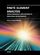Finite Element Analysis: Biomedical Apps to Industrial

This book presents represents a numerical technique for finding approximate solutions to partial differential equations as well as integral equations, permitting the numerical analysis of complex structures based on their material properties.

•Finite Element Analysis (David Moratal)

This book provides a bird's eye view on this very broad matter through original and innovative research studies exhibiting various investigation directions. You will gain a better understanding of where Finite Element Analysis stands today.

•Finite Difference Computing with PDEs: A Software Approach

This easy-to-read book introduces the basics of solving partial differential equations by means of finite difference methods. Unlike many of the traditional academic works on the topic, this book was written for practitioners.

•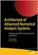Architecture of Advanced Numerical Analysis Systems

This unique open access book applies the functional OCaml programming language to numerical or computational weighted data science, engineering, and scientific applications. It's based on Owl, an OCaml-based numerical computing library.

•Tea Time Numerical Analysis: Experiences in Mathematics

This textbook was born of a desire to contribute a viable, free, introductory Numerical Analysis textbook. The ultimate goal of this book is to be a complete, one-semester, single-pdf, downloadable textbook designed for mathematics classes.

•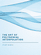The Art of Polynomial Interpolation (Stuart Murphy)

Exploring the techniques of interpolating data allows us to view the development and birth of a polynomial. This book is focused on laying a foundation for understanding and applying several common forms of Polynomial Interpolation.

•Numerical Algorithms: Computer Vision, Machine Learning, etc.

This book presents a new approach to numerical analysis for modern computer scientists, covers a wide range of topics - from numerical linear algebra to optimization and differential equations - focusing on real-world motivation and unifying themes.

•Numerical Methods and MATLAB Programming for Engineers

It covers the fundamentals while emphasizing the most essential numerical methods. Readers will enhance their programming skills using MATLAB to implement algorithms, discover how to use MATLAB to solve problems in science and engineering.

•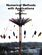Numerical Methods with Applications, 2nd Edition (Autar K Kaw)

This book entitled Numerical Methods with Applications is written primarily for engineering undergraduates taking a course in Numerical Methods. It offers a unique treatise to numerical methods which is based on a holistic approach and short chapters.

•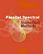Parallel Spectral Numerical Methods (Gong Chen, et al)

This book teaches the principals of Fourier spectral methods, their utility in solving partial differential equation and how to implement them in code. The programs will use Matlab and Fortran. A Python implementation of some of the Matlab programs is also provided.

•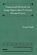Numerical Methods for Large Eigenvalue Problems (Yousef Saad)

This book is intended for researchers in applied mathematics and scientific computing as well as for practitioners interested in understanding the theory of numerical methods used for eigenvalue problems.

•First Semester in Numerical Analysis with Julia (Giray Okten)

This book presents the theory and methods, together with the implementation of the algorithms using the Julia programming language. The book covers computer arithmetic, root-finding, numerical quadrature and differentiation, and approximation theory.

•Numerical Analysis - Theory and Application (Jan Awrejcewicz)

This book is a book focused on introducing theoretical approaches of numerical analysis as well as applications of various numerical methods to either study or solving numerous theoretical and engineering problems.

Book Categories
 :All CategoriesTop Free BooksRecent BooksMiscellaneous BooksComputer EngineeringComputer LanguagesComputer ScienceData Science/DatabasesJava and Java EE (J2EE)Linux and UnixMathematicsMicrosoft and .NETMobile ComputingNetworking and CommunicationsSoftware EngineeringSpecial TopicsWeb Programming
Other Categories